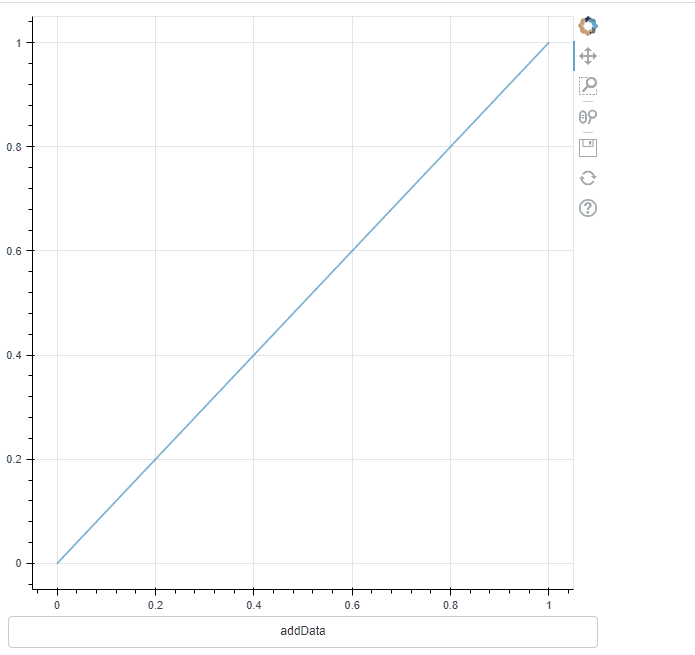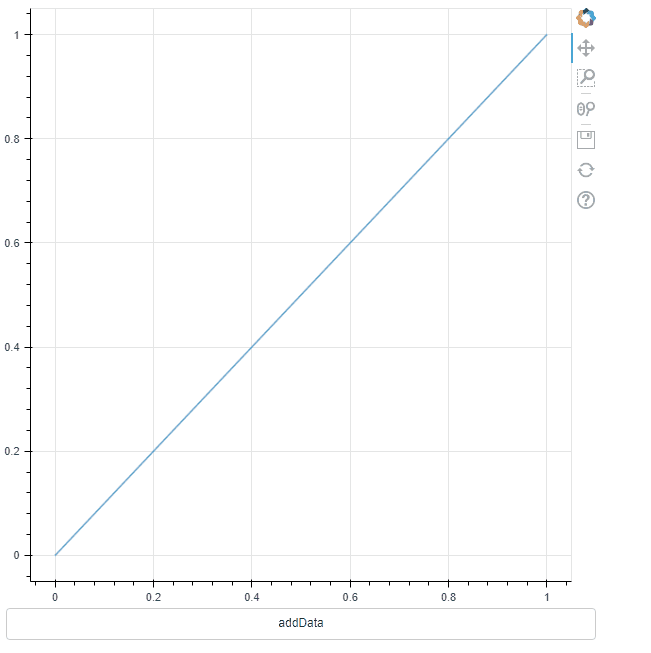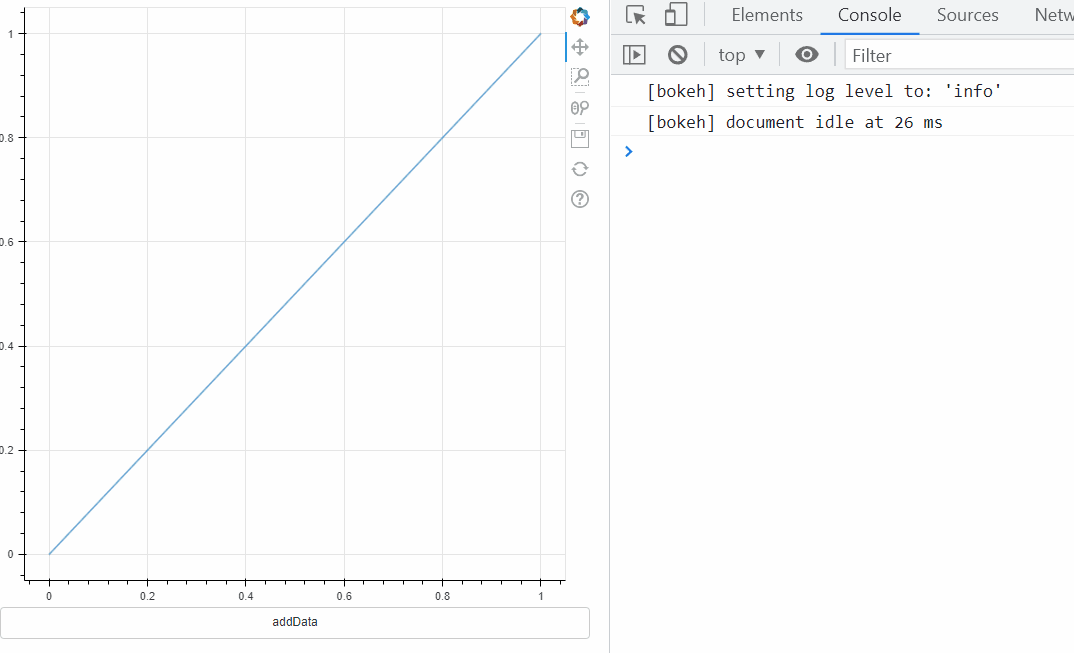# What are you trying to do?

I am trying to execute a CustomJS callback, or a series of callbacks, that will change the data of a CDS AND update the x_range/y_range bounds to fixed/set values. For my example I’ll just focus on x_range for simplicity:

The initialized x_range is “default”, i.e. a DataRange1d that will follow all renderers. The user may or may not, prior to triggering the callback, use pan/zoom and thereby hard-set the axis bounds. (Don’t think that matters but pointing it out just in case).

# What have you tried that did NOT work as expected?

I’ve got three very similar MRES:

1. Most basic → update data and x_range.start in the same callback:
``````from bokeh.plotting import figure,save
from bokeh.layouts import column
from bokeh.models import ColumnDataSource, CustomJS, Range1d, Button

src = ColumnDataSource(data={'x':[0,1],'y':[0,1]})

newdata = {'x':[0,1,2],'y':[2,3,5]}
f = figure()
r = f.line(x='x',y='y',source=src)

data_cb = CustomJS(args=dict(newdata=newdata,src=src,f=f)
,code='''
f.x_range.start = -5
src.data=newdata
'''
)

dbtn.js_on_click(data_cb)

save(column([f,dbtn]),'test.html')
``````

The cds.data update takes precedent, and the update to x_range.start is ignored.If you comment out the src.data=newdata line in data_cb, the f.x_range.start = -5 will be followed:1. Instantiate a Range1d object, pass it to the CustomJS, and update the figure’s x_range with it:
``````from bokeh.plotting import figure,save
from bokeh.layouts import column
from bokeh.models import ColumnDataSource, CustomJS, Range1d, Button

src = ColumnDataSource(data={'x':[0,1],'y':[0,1]})

newdata = {'x':[0,1,2],'y':[2,3,5]}
f = figure()
r = f.line(x='x',y='y',source=src)

xr = Range1d(start=-5,end=1)

data_cb = CustomJS(args=dict(newdata=newdata,src=src,f=f,xr=xr)
,code='''
f.x_range = xr
console.log(f.x_range.start)

//src.data=newdata
'''
)

dbtn.js_on_click(data_cb)

save(column([f,dbtn]),'test.html')
``````

This doesn’t work at all, even not bothering with the data update doesn’t trigger a re-render of the new range. The console.log does show that the figure’s x_range does get updated, but no re-render occurs! FYI I also tried a bunch .change.emit()s on the figure, the x_range, the figure’s properties etc.1. Make two callbacks:
``````from bokeh.plotting import figure,save
from bokeh.layouts import column
from bokeh.models import ColumnDataSource, CustomJS, Range1d, Button

src = ColumnDataSource(data={'x':[0,1],'y':[0,1]})

newdata = {'x':[0,1,2],'y':[2,3,5]}
f = figure()
r = f.line(x='x',y='y',source=src)

data_cb = CustomJS(args=dict(newdata=newdata,src=src,f=f)
,code='''
src.data=newdata
console.log(f.x_range.start)
'''
)
rng_cb = CustomJS(args=dict(f=f)
,code='''
f.x_range.start=-5
console.log(f.x_range.start)
'''
)

dbtn.js_on_click(data_cb)
dbtn.js_on_click(rng_cb)
save(column([f,dbtn]),'test.html')
``````

Result was same as 1.

Is this intended behaviour for reasons beyond me, is it a bug, and most importantly, is there a workaround?

Thanks…

1 Like

Thanks for the nice description with images. I have the same issue and still could not find a solution for that.

This topic was automatically closed 90 days after the last reply. New replies are no longer allowed.